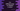# 8 Different ways to initialize a vector in C++## Different ways to initialize a vector:

Vector is similar to arrays. We can use it to hold data. But unlike arrays, we don’t have to initialize it with a size. The size of a vector increases automatically once we add an item to it.

So, we don’t have to worry about how many items we are adding to it. It will keep increasing the size.

There are different ways we can initialize a vector with content. In this post, we will learn how to initialize a Vector in C++ in different ways.

### Method 1: Initialize a vector and add elements to it:

We can create an empty vector of any type and by using the push_back method, we can add items to it.

push_back method takes an element as its argument and adds it to the end of a vector. It also increases the size of the vector by 1.

Let’s take a look at the below program:

``````#include <iostream>
#include <vector>

using namespace std;

int main()
{
vector<int> v;

v.push_back(1);
v.push_back(2);
v.push_back(3);

for (int i : v)
cout << i << endl;

return 0;
}``````

In this example,

• we created one int vector v
• By using push_back, we added three numbers to the end of this vector.
• The last for loop is printing the content of this vector.

If you run this program, it will print the below output:

``````1
2
3``````

### Method 2: Initialize with a size and default value:

We can also create a vector with a specific size and a default value. It will create one vector of that specific size and assign the given value to each of its elements.

For example:

``````#include <iostream>
#include <vector>

using namespace std;

int main()
{
int size = 4;
int value = -1;

vector<int> v(size, value);

for (int i : v)
cout << i << endl;

return 0;
}``````

In this example, the int vector v is created with a size 4 and each item of the vector is assigned -1.

The for loop is printing the content of the vector.

If you run this code, it will print:

``````-1
-1
-1
-1``````

### Method 3: Initialize with a size:

This is similar to the above example. You can initialize a vector with a predefined size. You need to pass the size, no need to pass value.

``````#include <iostream>
#include <vector>

using namespace std;

int main()
{
vector<int> v(10);

cout << v.size() << endl;

return 0;
}``````

It initializes a vector of size 10. If you run the program, it will print 10.

### Method 4: Initialize with values:

Vectors can be initialized like an array, i.e. we can initialize it with the values we want.

``````#include <iostream>
#include <vector>

using namespace std;

int main()
{
vector<int> v{1, 2, 3, 4, 5};

for (int i : v)
cout << i << endl;

return 0;
}``````

It is initialized with 1, 2, 3, 4 and 5.

If you run this program, it will print the below output:

``````1
2
3
4
5``````

### Method 5: Initialize one vector from another vector:

This is another way to initialize a vector. If we have a vector, we can create another vector from it.

``````#include <iostream>
#include <vector>

using namespace std;

int main()
{
vector<int> v{10, 20, 30, 40, 50};
vector<int> v2(v.begin(), v.end());

for (int i : v2)
cout << i << endl;

return 0;
}``````

It iterates through the vector v and creates the new vector v2. It will print the below output:

``````10
20
30
40
50``````

### Method 6: Copy a vector to another vector:

This method is similar to the above method. We can copy any vector to another vector.

``````#include <iostream>
#include <vector>

using namespace std;

int main()
{
vector<int> v{10, 20, 30, 40, 50};
vector<int> v2(v);

for (int i : v2)
cout << i << endl;

return 0;
}``````

It copied the content of v to v2.

``````10
20
30
40
50``````

### Method 7: Using iterator:

Iterator constructor can be used to construct a vector from an array. For example,

``````#include <iostream>
#include <vector>

using namespace std;

int main()
{
int arr[] = {10, 20, 30, 40, 50, 60};
std::vector<int> v(arr, arr + sizeof(arr) / sizeof(int));

for (int i : v)
cout << i << endl;

return 0;
}``````

It will print:

``````10
20
30
40
50
60``````

### Method 8: Use fill to fill a vector with a specific value:

fill method can be used to fill a vector with a specific value. This method is useful if you want to fill a vector with a value after the initialization.

``````#include <iostream>
#include <vector>

using namespace std;

int main()
{
vector<int> v(10);

fill(v.begin(), v.end(), -1);

for (int i : v)
cout << i << endl;

return 0;
}``````

It will assign -1 to each element of vector v.